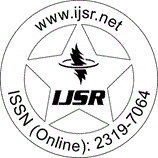International Journal of Science and Research (IJSR)
Call for Papers | Fully Refereed | Open Access | Double Blind Peer Reviewed

Downloads: 151 | Views: 201 | Weekly Hits: ⮙1 | Monthly Hits: ⮙1

Case Studies | Statistics | Indonesia | Volume 5 Issue 3, March 2016

# Spatial Variation in Infant Mortality with Geographically Weighted Poisson Regression (GWPR) Approach

Kristina Pestaria Sinaga  | Manuntun Hutahaean | Petrus Gea

Abstract: Regression analysis is a statistical analysis that aims to model the relationship between response variable and predictor variable. If the response variable distributes Poisson, the regression model used is Poisson regression. The main problem of this method is if the method is applied to spatial data. To overcome the spatial data problem, the statistical method to be used is Geographically Weighted Poisson Regression (GWPR) i. e. the local form of Poisson regression where the location noted. The results showed that the GWPR model parameters estimation used Maximum Likelihood Estimator (MLE) and was solved by using Newton-Raphson iteration. In this study the concept of geographical circumstances was applied to Poisson regression. The GWPR model application of the data percentage of infant mortality in North Sumatra, Indonesia showed that by using different weighting, the variables affecting the number of infant mortality per district/city in North Sumatra were also different. Based on the value of the Akaike Information Criterion (AIC) between Poisson regression model and GWPR model, it was known that GWPR model with weighting function of bisquare kernel was the better model used to analyze the number of infant mortality in North Sumatra Province in 2013 because it had the smallest AIC value.

Keywords: Infant Mortality, Poisson Regression, GWPR

Edition: Volume 5 Issue 3, March 2016,

Pages: 96 - 100